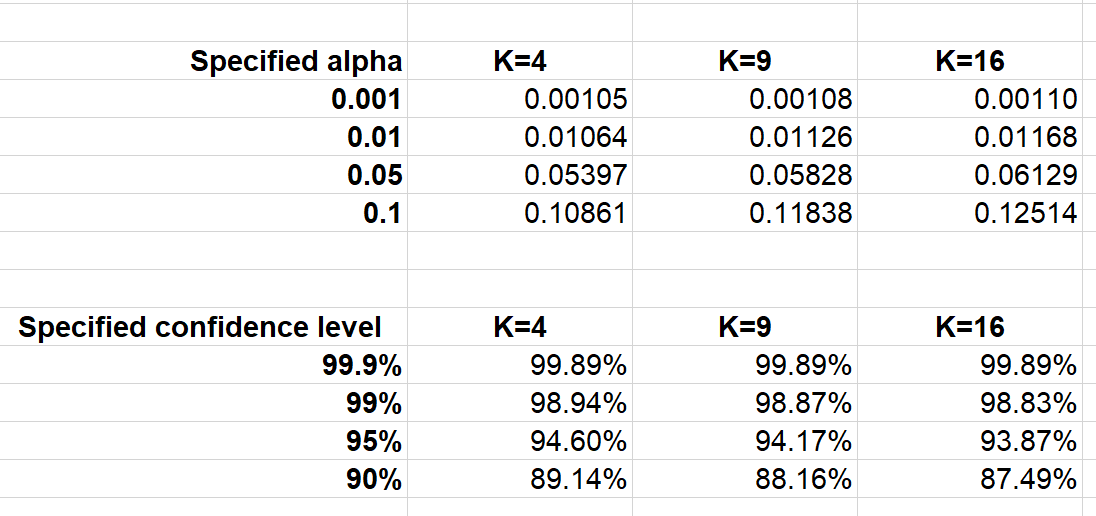KNOWLEDGEBASE - ARTICLE #2106

# Dunnett's test with an unequal sample size

When Prism, up to 7.04 and 7.0d, computes the Dunnett's multiple comparisons, the calculation of the P value depends on the number of treatment groups and the number of degrees of freedom (total sample size minus number of groups). This is the way that most texts show the test.

It turns out that this calculation is only 100% correct when all the treatment groups have the same sample size.

Since Dunnett's test is used to compare each treatment to the same control, it makes sense to have the control group larger than the others. Dunnett stated how to allocate the sample sizes most effectively(1). Make the ratio of the sample size for the control (Nc) to the sample size of the other groups (Ni) equal to the square root of the number of groups (K). Set Nc/Ni=sqr(K), where K is the total number of treatment groups excluding the control. So if you are comparing one control to four treatments, make the sample size of the control group twice as large as the treated groups (since K=4). Of course, that equation won't always work exactly since sample sizes are integers. But that is the goal. Dunnett didn't explain or prove that is the optimal sample size, but here is the proof.

If the sample sizes are not equal, the Dunnett's test performed by Prism (up to 7.04 and 7.0d) is not quite right. If the control has a larger sample size (as it should), the multiplicity adjusted P values from Prism were a tiny bit too low. If you don't look at the multiplicity adjusted P value but just at the decision of whether or not a difference was statistically significant, Prism was a bit too liberal about assigning "significance".

The discrepancy does not depend on sample size but does depend on the number of groups (K). We ran simulations only for the case recommended by Dunnett, where the sample size of the control equals the sample size of the other groups times the square root of K. For this case, here are the actual alpha values for when you choose alpha to be 0.001, 0.01 or 0.05, as well as the corresponding confidence levels.Excel file

If the control group has a smaller sample size than the treated groups (which would make no sense as an experimental design), the error goes the other way. Prism's P values would be a bit too large so Prism's designation of "significant" was a bit too conservative.

Starting with versions 7.05 (Windows) and 7.0e (mac), Prism correctly accounts for unequal sample size in Dunnett's test using the method explained in reference 1. Therefore, the results of Prism 7.05 and 7.0e and later will differ from the results of prior versions when sample sizes are unequal.

1, Dunnett, C. (1964). New tables for multiple comparisons with a control. Biometrics 20: 482–491.

Keywords: bug, unequal n, Dunnett Dunett Dunnet, Multiple comparisons

## Explore the Knowledgebase

Analyze, graph and present your scientific work easily with GraphPad Prism. No coding required.